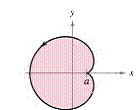Chapter 10.3, Problem 83E

Chapter
Section
Textbook Problem

# Areas of Simple Closed Curves In Exercises SI-86, use a computer algebra system and the result of Exercise 77 to match the closed curse with its area. (These exercises were based on “The Surveyor’s Area Formula'’ by Bart Braden, College Mathematics Journal, September 1986, pp. 335-337, by permission of the author.)(a) x 3 a b (b) x 8 π a 2 (c) 2 π a 2 (d) π a b (e) 2 π a b (f) 6 π a 2 Cardioid: ( 0 ≤ t ≤ 2 π ) x = 2 a cos t − a cos 2 t y = 2 a sin t − a sin 2 tTo determine

To Calculate: Match the area of the closed curve given by the parametric equation x=2acostacos2t and y=2asintasin2t on the interval 0t2π.

a)83ab     b)38πa2    c)2πa2d) πab    e)2πab     f)6πa2

Explanation

Given:

x=2acostacos2t and y=2asintasin2t 0t2π

Formula Used:

If y is a continuous function of x on the interval axb, where x=f(t) and y=g(t), then area of the curve in the interval axb is given by

abydx=t1t2g(t)f'(t)dt

Where, f(t1)=a, f(t2)=b and both g and f' are continuous on [t1,t2].

And,

ddxg(y)=ddyg(y)dydx

Calculation:

Differentiate x=2acostacos2t equation with respect to t,

dxdt=2asint+2asin2t

The area of curve will be given by:

=t1t2g(t)f(t)dt where x=f(t),y=g(t) and f(t1)=a,f(t2)=b

=02π2asintasin2t(2asint+2asin2t)dt

=02π(4a2sin2t+2a2sin2t

### Still sussing out bartleby?

Check out a sample textbook solution.

See a sample solution

#### The Solution to Your Study Problems

Bartleby provides explanations to thousands of textbook problems written by our experts, many with advanced degrees!

Get Started